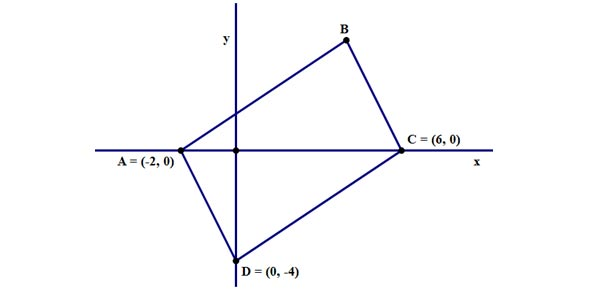# Vocabulary 7: Trigonometry And Coordinate Geometry (Not On Final)

24 Questions | Total Attempts: 23SettingsThis quiz covers 30 definitions important in trigonometry.  From the definition, you need to identify the correct term in your geometry vocabulary.  Spelling counts.  Good luck!

Related Topics
• 1.
In a right triangle, the sum of the squares of the measures of the legs equals the square of the measure of the hypotenuse.
• 2.
An angle formed by a horizontal line and the line of sight to an object below the level of the horizontal.
• 3.
An angle formed by a horizontal line and the line of sight to an object above the level of the horizontal.
• 4.
For an acute angle of a right triangle, the ratio of the length of the leg adjacent to the acute angle to the length of the hypotenuse.
• 5.
For any positive numbers a and b, the positive number x such that a/x = x/b.
• 6.
For any triangle where a, b and c represent the measures of sides opposite angles with measures A, B, and C, respectively:             a2 = b2 + c2 - 2bc cosA            b2 = a2 + c2 – 2ac cosB   and             c2 = a2 + b2 – 2ab cosC
• 7.
For any triangle where a, b and c represent the measures of sides opposite angles with measures A, B, and C, respectively: sinA/a = sinB/b = sinC/c
• 8.
A set of numbers a, b, and c such that a2 = b2 + c2.
• 9.
For an acute angle of a right triangle, the ratio of the length of the leg opposite the acute angle to the length of the hypotenuse.
• 10.
Finding the measures of all the angles and sides of a triangle.
• 11.
For an acute angle of a right triangle, the ratio of the length of the leg opposite the acute angle to the length of the leg adjacent to the acute angle.
• 12.
The study of the properties of triangles and trigonometric functions and their applications.
• 13.
In the proportion a/b = c/d, the values ad and bc.  The proportion is true if and only of these are equal.
• 14.
In the proportion a/b = c/d, the values b and c.
• 15.
A relationship between input and output, in which one or more operations are performed on the input to get the output, and there is exactly one output for each input.
• 16.
A technique for graphing a linear equation by locating the x- and y-intercepts
• 17.
An equation that can be written in the form Ax + By = C, where A, B and C are real numbers, with A and B not both 0.
• 18.
A pair of numbers given in a specific order, used to locate points in a plane.
• 19.
Three numbers given in a specific order, used to locate points in three dimensional space.
• 20.
The form of equations given as a/b = c/d, that states that two ratios are equivalent.
• 21.
A comparison of two numbers using division.
• 22.
For linear equations, an equation that can be written in the form Ax + By = C, where A, B and C are real numbers, with A and B not both 0.
• 23.
Two sets of data plotted as ordered pairs in a coordinate plane.
• 24.
A group of two or more equations with the same variables.